# How to Calculate and Solve for Gravitational Potential | The Calculator Encyclopedia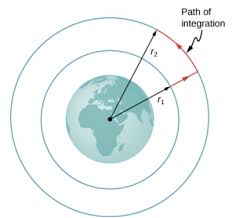The image above represents the Gravitational potential.

To compute the gravitational potential of a field, two essential parameters are needed which are mass (m) and radius (r).

The formula for calculating the gravitational potential;

V = (Gm) / r

Where;
V = Gravitational potential
m = Mass

Let’s solve an example;
Find the gravitational potential of a field when the mass is 14 cm with a radius of 9 cm.

This implies that;
m = Mass = 14 cm
r = Radius = 9 cm

V = (Gm) / r
V = (6.67 x 10-11 x 14) / 9
V = 1.0375e-10 / 9
V = 1.0375e-10

Therefore, the gravitational potential is 1.0375e-10 Volts (V).

Nickzom Calculator – The Calculator Encyclopedia is capable of calculating the gravitational potential.

To get the answer and workings of the gravitational potential using the Nickzom Calculator – The Calculator Encyclopedia. First, you need to obtain the app.

You can get this app via any of these means:

To get access to the professional version via web, you need to register and subscribe for NGN 1,500 per annum to have utter access to all functionalities.
You can also try the demo version via https://www.nickzom.org/calculator

Once, you have obtained the calculator encyclopedia app, proceed to the Calculator Map, then click on Gravitational Field under the Physics section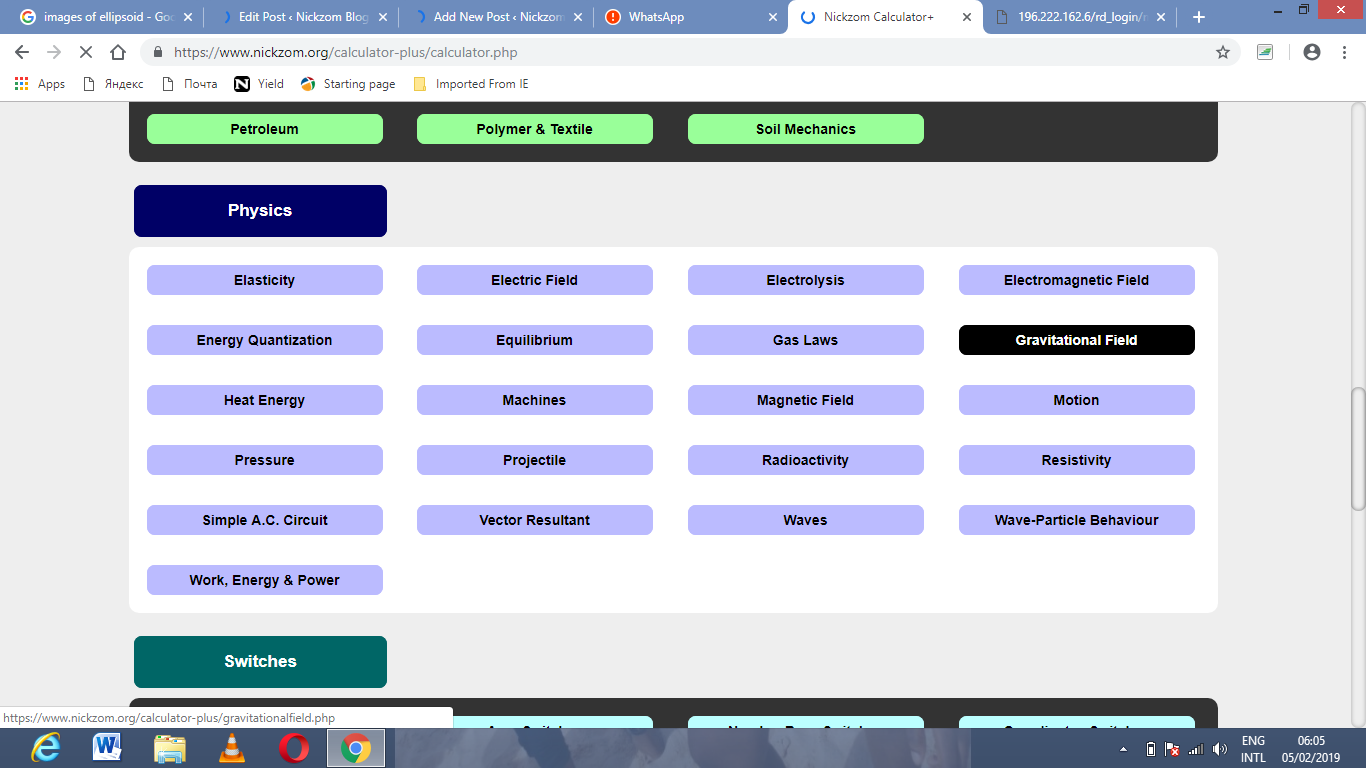Now, Click on Gravitational Potential under Gravitational FieldThe screenshot below displays the page or activity to enter your values, to get the answer for the gravitational potential according to the respective parameters which are the mass (m) and radius (r).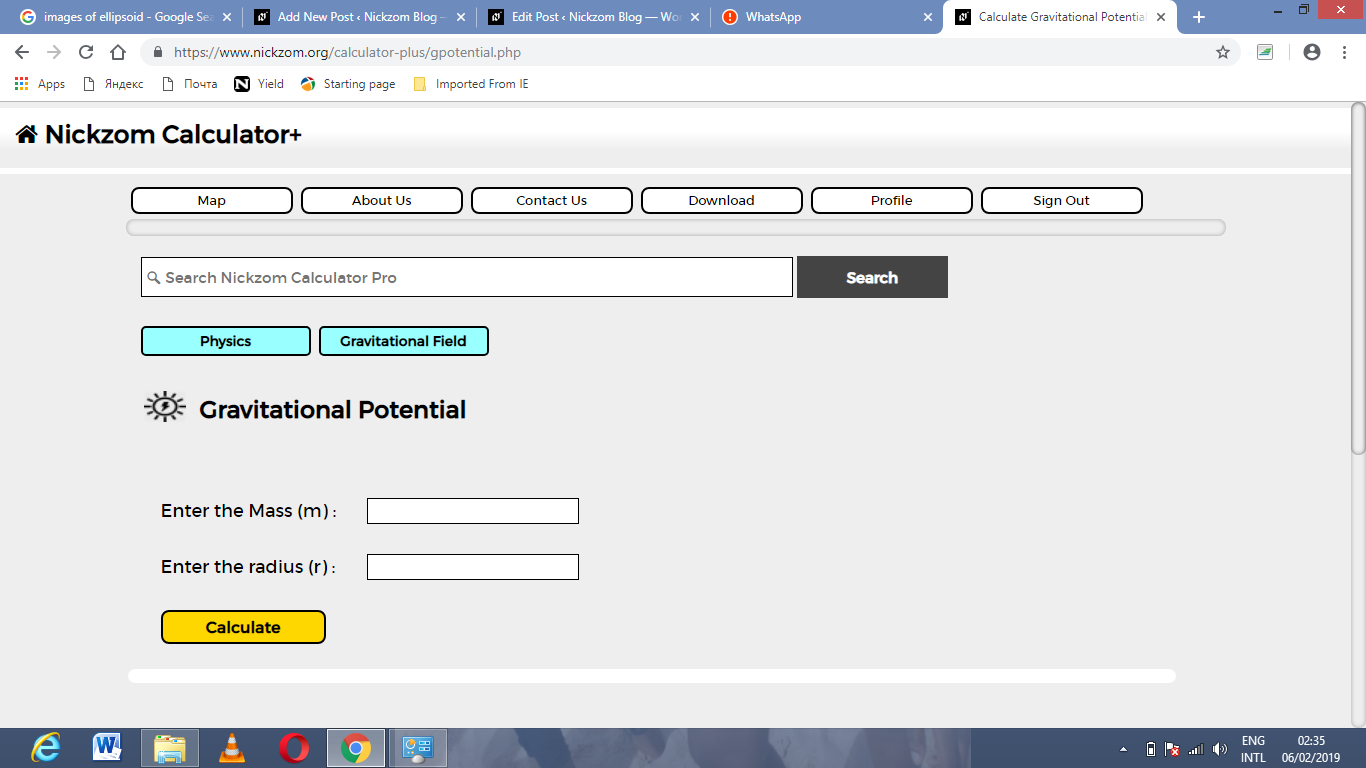Now, enter the values appropriately and accordingly for the parameters as required by the example above where the mass (m) is 14 cm  and radius (r) is 9 cm.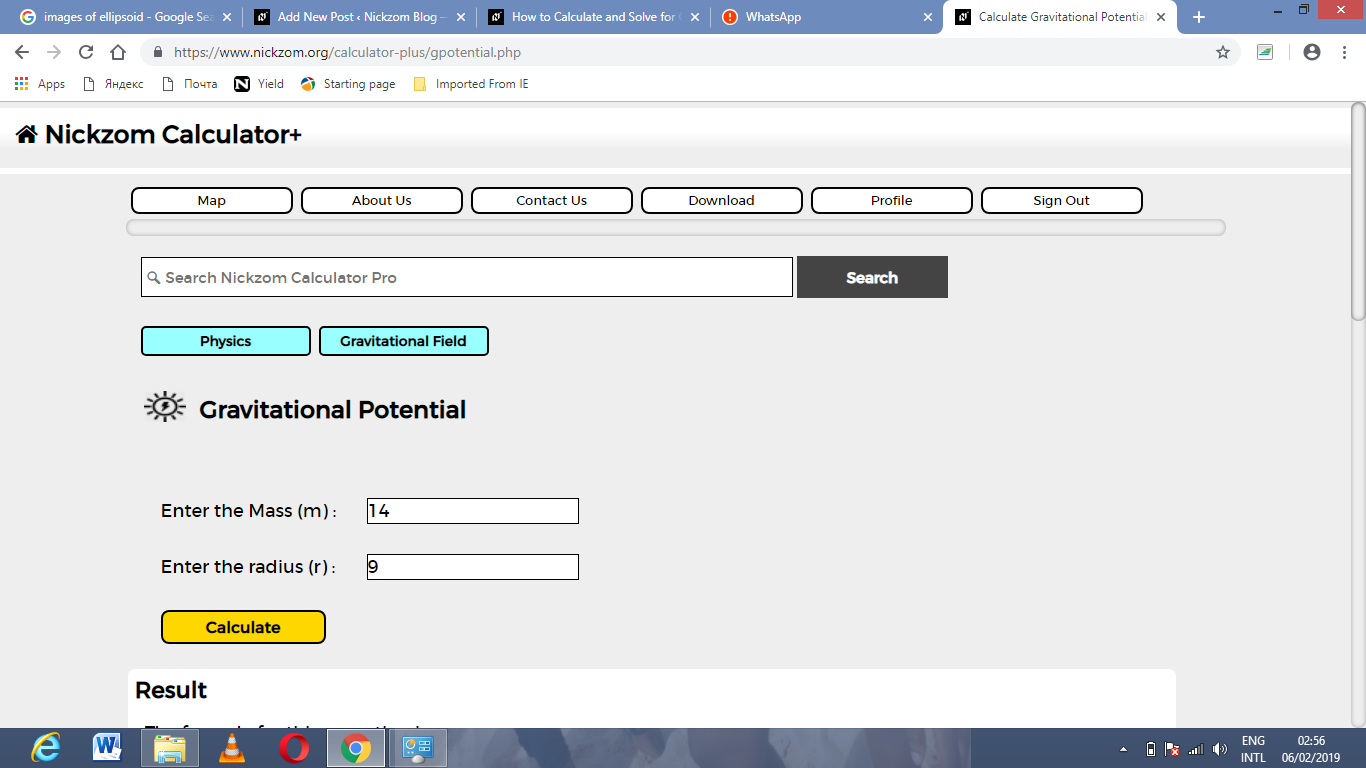Finally, Click on Calculate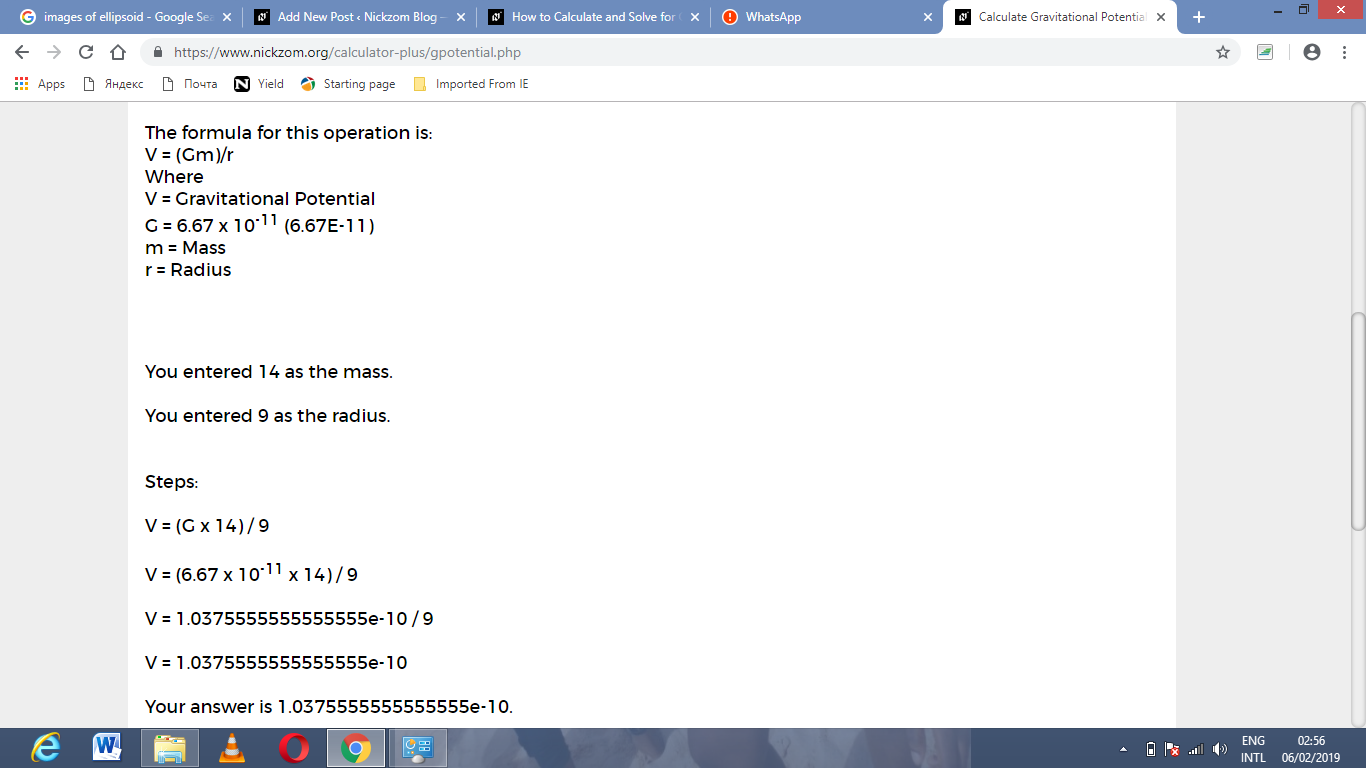As you can see from the screenshot above, Nickzom Calculator – The Calculator Encyclopedia solves for the gravitational potential and presents the formula, workings and steps too.